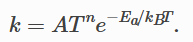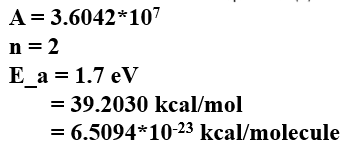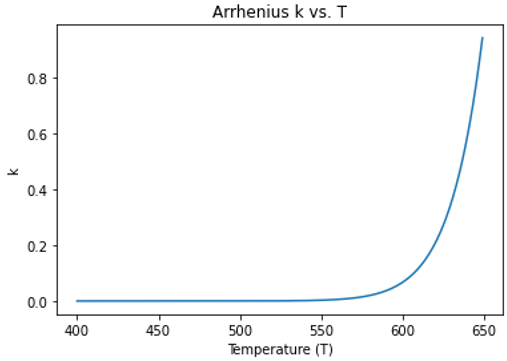# Arrhenius equation in fix bond/react command

Hi there,

I have some confusing issues with the Arrhenius constraints in fix bond/react command. I will describe my problem in as much detail as possible.

The official Lammps documentation for this constraint is as follows，

My doubt is how are A and n in the formula determined? Besides, the unit of k is related to the order of a chemical reaction. So why can the k value and the chemical reaction probability be linked in the formula?

Any help on the issues would be greatly appreciated!

Thanks,

Zilin

@jrgissing, the author of fix bond/react, should be able to provide some explanations.

As background, this constraint is meant as a way to incorporate local temperature and an effective activation energy into reactions. This idea, including assuming k is proportional to reaction probability, comes from Monte Carlo simulations.

As far as determining A and n (n is zero for the standard Arrhenius equation), that is a system-specific question because A is determined empirically. A few pointers include that A for simulations is not quantitatively comparable to experiment. Instead, it should be used to scale the Arrhenius equation between 0 and 1 for the temperature range of interest. I highly encourage plotting this function! This constraint is typically most useful for simulations that have differences in temperature, either in space or in time. For example, it could be used to activate a polymerization reaction at a certain temperature.

Okay, thank you for your suggestion.

I still have a question.

I tried to plot the Arrhenius equation. According to lammps official website documentation, Ea is the activation energy (units of energy). I thought that the energy unit here is related to the units defined in the lammp input file (for example, my units are real, so the energy unit should be kcal/mol). But the formula defined in the manual is as follows,I saw on Wikipedia that when the denominator is kB, the unit of Ea on the numerator should be energy per molecule instead of kcal/mol. Based on this, I defined the following parameters and plotted the Arrhenius equation,My map file is,

According to the Arrhenius diagram above, can it be considered that chemical reactions are possible when the temperature is 600-650 K?

Yes, no reactions will occur when k is zero, all eligible reactions will occur when k is >= 1, and an eligible reaction will have a 50% chance of occuring when k = 0.5, etc. That is a good point about the units, this feature was partly borrowed from DPD, which typically uses ‘metal’ units, but it might make more sense to use the gas constant R in the Arrhenius equation if it is mostly being used for atomistic simulations, i.e., ‘real’ units.

Thank you very much, sir. I learned a lot of great things from the discussions with you.

Sir, I found a slide you wrote about REACTER, one of the pages is like this，

In the picture on the left of the slide, the unit of Ea is kcal/mol, but the denominator is still KB. Does this mean that there is no need to consider too many details in the current practical application. In other words, when the unit is real, it is also ok to use KB directly? (Sorry, I tried to look at the source code on github, but I couldn’t find the corresponding formula due to my poor programming knowledge.)

Sir, another question is how the picture on the right side of the above slide is made? I don’t seem to have noticed that the number of times the reaction occurs can be counted in the command.

Finally, thank you very much for your time and patient guidance.

Best wishes,
Zilin

Yes, to be clear, LAMMPS specifies k_B in units of kcal/(mol*K) when using ‘real’ units, making it equivalent to the gas constant. So, your above analysis of which units to use for E_a may be incorrect. LAMMPS units specifications, including k_B, can be found in ‘update.cpp.’ The relevant calculation of hte Arrhenius equation within ‘fix bond/react’ can be found by searching for ‘force->boltz’ within the ‘check_constraints’ routine, for example.

The cumulative number of reactions that have occurred (for each reaction) is output by ‘fix bond/react’ as a vector. Examples of this output can be found with the examples provided in LAMMPS here: /examples/PACKAGES/reaction

I see.
Thank you so much for your patience. To be honest, although REACTER is a bit difficult to learn, it is really cool !!! (I still need to spend some time figuring out some details.)

May the joy and happiness around you today and always,

Zilin

Sir, Regarding Arrhenius constraints, I would like to ask you another question.

I want to output the instantaneous temperature at the reaction site when each reaction occurs. I then wrote the following input script:

``````fix           rxns all bond/react stabilization yes statted_grp .03 &
react rxn1_stp1 all 1 0.0 3 mol1 mol2 rxn1_stp1.map

compute       chunk_atom all chunk/atom f_rxns
compute       chunk_temp all temp/chunk chunk_atom temp
thermo_style    custom step atoms temp epair etotal press density c_chunk_temp f_rxns
``````

But the result is wrong:
ERROR: Compute chunk/atom fix does not calculate per-atom values (…/compute_chunk_atom.cpp:368)

So is it not possible to output the instantaneous temperature at the reaction site each time a reaction occurs?

Best wishes,
Zilin

It looks like your syntax for ‘compute chunk/atom’ is incorrect.
Please review the documentation: compute chunk/atom command — LAMMPS documentation

No, ‘fix bond/react’ currently does not print out this information, although it would require just a small modification of the source code to do so. The temperature is calculated by the ‘get_temperature’ routine

Thank you, sir.

I wish you have a nice day.